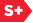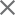### Proceedings Paper

Modeling and estimation of wavelet coefficients using elliptically-contoured multivariate laplace vectors
Author(s): Ivan W. Selesnick
Format Member Price Non-Member Price
PDF \$17.00 \$21.00

Paper Abstract

In this paper, we are interested in modeling groups of wavelet coefficients using a zero-mean, elliptically-contoured multivariate Laplace probability distribution function (pdf). Specifically, we are interested in the problem of estimating a d-point Laplace vector, s, in additive white Gaussian noise (AWGN), n, from an observation, y = s + n. In the scalar case (d = 1), the MAP and MMSE estimators are already known; and in the vector case (d > 1), the MAP estimator can be obtained by an iterative successive substitution algorithm. For the special case where the contour of the Laplace pdf is spherical, the MMSE estimators for the vector case (d > 1) have been derived in our previous work; we have shown that the MMSE estimator can be expressed in terms of the generalized incomplete Gamma function. For the general elliptically-contoured case, the MMSE estimator can not be expressed as such. In this paper, we therefore investigate approximations to the MMSE estimator of a Laplace vector in AWGN.

Paper Details

Date Published: 20 September 2007
PDF: 15 pages
Proc. SPIE 6701, Wavelets XII, 67011K (20 September 2007); doi: 10.1117/12.736047
Show Author Affiliations
Ivan W. Selesnick, Polytechnic Univ. (United States)

Published in SPIE Proceedings Vol. 6701:
Wavelets XII
Dimitri Van De Ville; Vivek K. Goyal; Manos Papadakis, Editor(s)